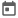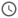# Verilog Arrays##### 04 Aug 2021##### 3 mins

Array means an ordered list of variables. In Verilog, we can have an any dimension of array and can be of 2 types, i.e., scalar and vector. Arrays are only allowed for net, reg, integer and real data types. Array elements are located with the help of index value. These index value starts with 0 and thus the last index = size_of_array-1.

### Scalar vs Vector Array

Scalar Array is an array of one 1-bit data type. `Ex – reg a[3:0]` - This is an array of size 4 and where each element can hold 1-bit data. Vector Array is an array of vectors. As we have seen earlier vectors are nothing but a group of 1-bit data types combined to increase the size of data type. `Ex – reg [3:0] a [7:0]` - This is an array of size 8, where each element can hold 4-bit reg data.

### Dimension of array

Dimension can be related to dimensions of real objects, where 1-D would mean that object will just have width, 2-D means it will have length and width and 3-D means it will have height, length, and width. But for real life objects dimensions are related. In Verilog, array can be of any dimension and would simply mean that how many index variables are required to access a particular element of the array.

#### Example

``````module multi_array;
// 1-D array - only one index variable will be required to access a element
reg a [7:0]; // a scalar array having size = 8

// 2-D array - 2 index variables will be required
reg b [3:0][7:0]; // a scalar 2-D array having rows = 4, column = 8

// 3-D array - 3 index variables will be required
reg c [1:0][3:0][7:0]; // a scalar 3-D array having height = 2, rows = 4, column = 8
endmodule``````

### Memories

Memories are a vital part of hardware devices as it stores data. Memories have 2 important terms `width` and `depth`. Width represents the no. of bits each element can store, and depth is the number of elements a memory can store.

If we see memories in terms of array, then a 1-D vector/scalar array represents a memory.

#### Example

``````module memory;
// mem is a memory of width 8 and depth 256.
// as index element can have a value from 0 - (2^8)-1
reg [7:0] mem [7:0];
endmodule``````

### Accessing array elements

All array elements cannot be accessed at the same time. Thus, while reading or writing the data from array elements, we must access array elements one at a time, using loops. Generally, we use `for` loop for this purpose, but at times other types of loops can also be used.

As mentioned earlier, no. of index variable depends upon the dimension of array and no. of loops is equal to no. of index variable.

#### Example

``````module array_example;
// mem is a memory of width 8 and depth 256.
// as index element can have a value from 0 - (2^8)-1
reg [7:0] arr [3:0][7:0];

integer i, j; // 2 index variables as this is 2-D array
initial begin
for(i=0;i<4;i=i+1) begin
for(j=0;j<8;j=j+1) begin
arr[i][j] = i*j;
end
end

// displaying the array
\$display("Array elements are: ");
for(i=0;i<4;i=i+1) begin
for(j=0;j<8;j=j+1) begin
\$write("%0d\t", arr[i][j]);
end
\$display("");
end
end
endmodule``````
##### Output
``````# 0	0	0	0	0	0	0	0
# 0	1	2	3	4	5	6	7
# 0	2	4	6	8	10	12	14
# 0	3	6	9	12	15	18	21``````